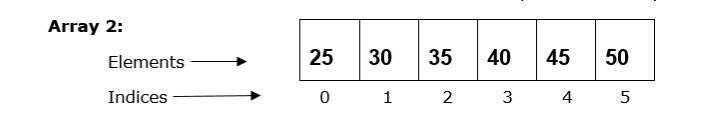# How to add an element to an Array in Java?

Array is a linear data structure that is used to store a group of elements with similar datatypes. It stores data in a sequential manner. Once we create an array we can’t change its size i.e. it is of fixed length. Adding an element to a given array is a vastly common operation. In this article, we will discuss how to add an element to an Array through Java example programs.

## Adding an element to an Array in Java

Let’s understand the operation with an example first −We will add a new element ‘50’ at the end of above array. The new array would become:### Syntax for Array

Data_Type nameOfarray[] = {values separated with comma};


### Approach 1

• Create an array of integer type and store its length to an integer variable

• Now, create another array of integer type but one size larger than the previous array.

• Moving further copy the elements of first element to the second array using a for loop and also, add a new element after copying

• In the end, print the new element using another for loop.

### In the following example, we will add an element to the given array by using the for loop.

import java.util.*;
public class Increment {
public static void main(String[] args) {
int aray[] = {25, 30, 35, 40, 45};
int sz = aray.length;
System.out.print("The given array: ");

// to print the older array
for(int i = 0; i < sz; i++) {
System.out.print(aray[i] + " ");
}
System.out.println();
int newAray[] = new int[sz + 1];
int elem = 50;

// to append the element
for(int i = 0; i < sz; i++) {
newAray[i] = aray[i]; // copying element
}
newAray[sz] = elem;
System.out.print("The new array after appending the element: ");

// to print new array
for(int i = 0; i < newAray.length; i++) {
System.out.print(newAray[i] + " ");
}
}
}


#### Output

The given array: 25 30 35 40 45
The new array after appending the element: 25 30 35 40 45 50


### Approach 2

• Create an array of Integer type. Here, ‘Integer’ is a wrapper class. Using for loop display the array.

• Now, define an ArrayList with the previous array using ‘Arrays.asList()’ method.This method takes an array as an argument and returns it as a List.

• Moving further add a new element to the ArrayList using a built-in method ‘add()’.

• Display the result and exit.

### In the following example, we will add an element to the given array by using the ArrayList.

import java.util.*;
public class Increment {
public static void main(String[] args) {
Integer aray[] = {25, 30, 35, 40, 45};
int sz = aray.length;
System.out.print("The given array: ");

for(int i = 0; i < sz; i++) {
System.out.print(aray[i] + " ");
}
System.out.println();

// creating an ArrayList with old array
ArrayList<Integer> arayList = new
ArrayList<Integer>(Arrays.asList(aray));
System.out.print("The new array after appending the element: " + arayList);
}
}


#### Output

The given array: 25 30 35 40 45
The new array after appending the element: [25, 30, 35, 40, 45, 50]


## Conclusion

Unlike other programming languages, Java does not provide any in-built methods for appending elements to an array. The reason is that array is of fixed size. Therefore, we need to put our custom logic to add an element to an array.

Updated on: 20-Jul-2023

5K+ Views